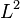# Linear regression

## Summary

Item Value
Type of variable predicted Continuous; however this could also be used to predict discrete variables that can be placed on a continuum, such as values that are constrained to be integers.
Format of prediction A point estimate of the value is output
Functional form of model Computes the point estimate for the value being predicted by taking a linear combination of the features. The coefficients for the linear combination are the unknown parameters (also known as model weights) that need to be determined by the learning algorithm.
Typical cost function The most typical is ordinary least squares (OLS) regression, where the loss associated with each prediction is the square of the distance between the prediction and the actual value. There are many variants, such as weighted least squares (where different predictions get different weights), total least squares (where errors in both dependent and independent variables are modeled), non-negative least squares (where the parameters are all constrained to be non-negative).
Typical regularization choices$L^1$ (lasso),$L^2$ (ridge), and a mix (elastic net). Other Bayesian priors may also be used to generate regularizations.